# A Bayesian Approach

### Home | Academic Articles

Purpose

The purpose of constructing a confidence interval for proportions using a Bayesian approach is reduce the margin of error from the Frequentist school confidence interval for proportions.

Example

To illustrate, suppose a store stocks boxes of Brand X cereal each day so that there are 100 boxes in total. On day 1, they sell 62 boxes. What is the 95% confidence interval of the percentage of boxes sold per day?

Using the Frequentist school approach, the interval would be: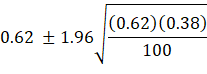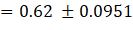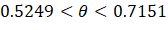The width of the interval is 0.7151 – 0.5249 = 0.1902. Nice and simple.

Now, to use the Bayesian approach, we need a prior distribution for θ. Since we don’t have any prior distribution, we can use the uniform distribution in which P(θ) = 1.

However, the uniform distribution is a special case of the Beta distribution in which α = 1 and β = 1. As is well documented in the literature, the Beta distribution is the conjugate prior of the binomial distribution.

To find the posterior distribution for θ, we combine the posterior distribution with the current data in which n represents the total number of cases and x represents the number of successes. When we do this, the posterior distribution of θ follows a Beta distribution with α = x + α and β = n + β – x.

In our example, α = 62 + 1 = 63 and β = 100 + 1 – 62 = 39. Note that the sum of α and β is 102. This is due to the sum of n = 100, the prior α = 1 and the prior β = 1.

To construct the 95% confidence interval using the Beta distribution, we need the 2.5th and 97.5th percentiles of the Beta distribution with α = 63 and β = 39. For this distribution, the 2.5th percentile is 0.5218 and the 97.5th percentile is 0.7091. Thus, the interval is: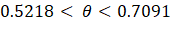The width of this interval is 0.7091 – 0.5218 = 0.1873 which is a tad tighter than the 0.1902 from the previous confidence interval.

Suppose that over the next 4 days, as on the first day, there are 100 boxes on the shelf and these are the number of boxes sold:

 Day 2 Day 3 Day 4 Day 5 28 8 42 65

If we sum the number of sales over the 5 days, p = 205/500 = 0.41. Then the 95% confidence interval from the frequentist school is: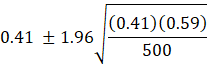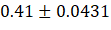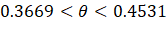The width of this interval is 0.4531 – 0.3669 = 0.0862.

Using the Beta distribution, we find the new values of α and β:

 Prior Day 2 Day 3 Day 4 Day 5 Total α 63 28 8 42 65 206 β 39 72 92 58 35 296

Note that we use α = 63 and β = 39 as the new priors. This time, the 2.5th percentile is 0.3677 and the 97.5th percentile is 0.4537. The width of this interval is 0.4537 – 0.3677 = 0.086. The width is still slightly less than that of 0.0862 but the difference is narrowing. However, as explained in the technical details section, the Bayesian confidence interval will always be narrower than the frequentist school confidence interval, provided the total number of cases is greater than 3.

Technical details

The formula for the Beta distribution is: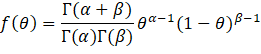In the formula,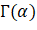represents the gamma function of α. The formula for the gamma function is: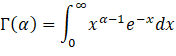If α is a whole number, then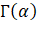is equal to (α – 1)!. For example, if α = 3, then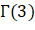= 2! = 2 x 1 = 2. It should be noted that in Excel, the factorial function is called fact. So, in an Excel spreadsheet, to call 2!, you would type in a cell =fact(2) to get the result of 2.

For example,1 with α = 1 and β = 1, we have: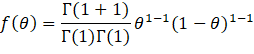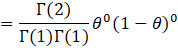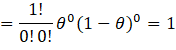This is due to the fact that 0! = 1 by definition.

To eventually construct the posterior distribution of θ, we can say: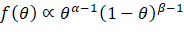The formula for the binomial distribution is: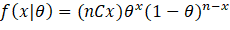In the formula, nCx is the number of ways to choose x items from n. Its formula is: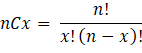In order to construct the posterior distribution of θ, we can say: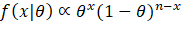In stats speak, f(x | θ) is called the likelihood.

To construct the posterior distribution of θ, we combine the prior and likelihood: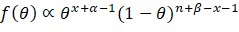If there are a small number of cases or successes, the Frequentist school has a tool at its disposal: Wilson’s estimate. In using this tool, the sample proportion used in the interval is: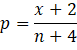This raises the question as to why Bayesian analysis is better when there are a small number of successes.

Let’s start with the mean and variance of the Beta distribution.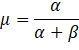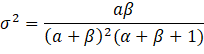In the case of the posterior distribution of θ in which α = x + α and β = n + β – x, the mean and variance are: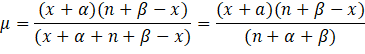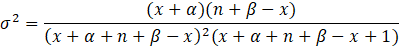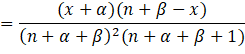Let’s examine the case in which the prior is the uniform distribution in which α = 1 and β = 1. The mean and variance become: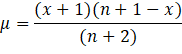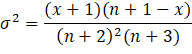If we take x equal to zero, the mean and variance become: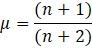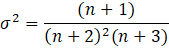If we examine the variance of θ using Wilson’s estimate, we have: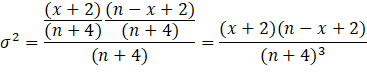If we take x equal to zero, we have: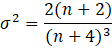As is well documented, the variance (or standard deviation) has an effect on the width of a confidence interval: A larger variance results in a wider confidence interval.

This raises the question: For which values of n is the variance of the Beta distribution less than that using Wilson’s estimate?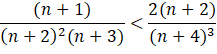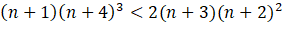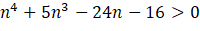The above inequality holds once n > 2. If x is increased to 1, then the variance of the Beta distribution is less once n > 3.